• ## 最大化期望算法（EM）详解

万次阅读 多人点赞 2018-07-30 09:15:53
我们知道最大似然估计的根本目的是根据抽样的到的样本（即数据），反推出最有...这个时候就要依靠最大化期望（EM）算法了。 简单的说，EM算法是在依赖于无法观测的隐藏变量的概率模型中，寻找参数最大似然估计或者...
我们知道最大似然估计的根本目的是根据抽样的到的样本（即数据），反推出最有可能的分布参数（即模型），这是一个非常典型的机器学习的思想。所以在很多领域最大似然估计有着极为广泛的应用。然而，如果已知的数据中含有某些无法观测的隐藏变量时，直接使用最大似然估计是不足以解决问题的。这个时候就要依靠最大化期望（EM）算法了。

简单的说，EM算法是在依赖于无法观测的隐藏变量的概率模型中，寻找参数最大似然估计或者最大后验估计的算法。

1. 最大似然估计

最大似然其实基本的原理非常简单，假设我们手里现在有一个样本，这个样本服从某种分布，而分布有参数，可如果我现在不知道这个样本分布的具体参数是多少，我们就想要通过抽样得到的样本进行分析，从而估计出一个较准确的相关参数。

以上，这种通过抽样结果反推分布参数的方法就是“最大似然估计”。现在简单思考一下怎么去估计：已知的一个抽样结果和可能的分布（比如说高斯分布），那我就像小学生解方程那样呗，先设出分布的参数（比如高斯分布中就是设出σ$\sigma$$\sigma$和μ$\mu$$\mu$），然后我计算得到现在这个抽样数据的概率函数，令这个概率最大，看此时相关参数的取值。

这个思路很容易理解，能使得概率最大的参数一定是“最可能”的那个，这里的“最可能”也就是最大似然估计中“最大似然”的真正含义。

只是这么说可能有点抽象，看一个具体的例子。设产品有合格、不合格两类，未知的是不合格品的概率p$p$$p$，显然这是一个典型的两点分布b(1,p)$b\left(1,p\right)$$b(1, p)$。我们用随机变量X$X$$X$表示是否合格，X=0$X=0$$X = 0$表示合格，X=1$X=1$$X = 1$表示不合格。如果现在得到了一组抽样数据(x1,x2,…,xn)$\left({x}_{1},{x}_{2},\dots ,{x}_{n}\right)$$(x_1, x_2, \dots, x_n)$，那么不难写出抽样得到这组数据的概率：

f(X1=x1,X2=x2,…,Xn=xn;p)=∏i=1npxi(1−p)1−xi(1)$\begin{array}{}\text{(1)}& f\left({X}_{1}={x}_{1},{X}_{2}={x}_{2},\dots ,{X}_{n}={x}_{n};p\right)=\prod _{i=1}^{n}{p}^{{x}_{i}}\left(1-p{\right)}^{1-{x}_{i}}\end{array}$\begin{equation}
f(X_1 = x_1, X_2 = x_2, \dots, X_n = x_n; p) =  \prod_{i = 1}^n p^{x_i}(1 - p)^{1 - x_i}
\end{equation}

我们把上面这个联合概率叫做样本的似然函数，一般把它两侧同时取对数（记为对数似然函数L(θ)$L\left(\theta \right)$$L(\theta)$）。L(θ)$L\left(\theta \right)$$L(\theta)$关于p$p$$p$的求偏导数，令偏导数为0，即可求得使得L(p)$L\left(p\right)$$L(p)$最大的p$p$$p$值。

∂L(p)∂p=0⇒p^=∑i=1nxi/n(2)$\begin{array}{}\text{(2)}& \frac{\mathrm{\partial }L\left(p\right)}{\mathrm{\partial }p}=0⇒\stackrel{^}{p}=\sum _{i=1}^{n}{x}_{i}/n\end{array}$\begin{equation}
\frac{\partial L(p)}{\partial p} = 0 \Rightarrow \hat{p} = \sum_{i = 1}^{n} x_i / n
\end{equation}
其中，求得的p$p$$p$值称为p$p$$p$的最大似然估计，为示区分，用p^$\stackrel{^}{p}$$\hat{p}$表示。

其他分布可能计算过程更加复杂，然而基本的步骤与这个例子是一致的。我们总结一下：设总体的概率函数为p(x;θ)$p\left(x;\theta \right)$$p(x; \theta)$，θ$\theta$$\theta$为一个未知的参数，现已知来自总体的一个样本x1,x2,…,xn${x}_{1},{x}_{2},\dots ,{x}_{n}$$x_1, x_2, \dots, x_n$那么求取θ$\theta$$\theta$的最大似然估计的步骤如下：

写出似然函数L(θ)$L\left(\theta \right)$$L(\theta)$，它实际上就是样本的联合概率函数
L(θ)=p(x1;θ)⋅p(x2;θ)⋅…p(xn;θ)(3)$\begin{array}{}\text{(3)}& L\left(\theta \right)=p\left({x}_{1};\theta \right)\cdot p\left({x}_{2};\theta \right)\cdot \dots p\left({x}_{n};\theta \right)\end{array}$\begin{equation}
L(\theta) = p(x_1; \theta) \cdot p(x_2; \theta) \cdot \dots p(x_n; \theta)
\end{equation}
对似然函数求取对数，并整理
ln(L(θ))=lnp(x1;θ)+⋯+lnp(xn;θ)(4)$\begin{array}{}\text{(4)}& \mathrm{ln}\left(L\left(\theta \right)\right)=\mathrm{ln}p\left({x}_{1};\theta \right)+\cdots +\mathrm{ln}p\left({x}_{n};\theta \right)\end{array}$\begin{equation}
\ln(L(\theta)) = \ln p(x_1; \theta) + \dots + \ln p(x_n; \theta)
\end{equation}
关于参数θ$\theta$$\theta$求偏导，并令偏导数为0，解得参数θ^$\stackrel{^}{\theta }$$\hat{\theta}$，这就是参数θ$\theta$$\theta$的最大似然估计
∂L(θ)∂θ=0⇒θ^=…(5)$\begin{array}{}\text{(5)}& \frac{\mathrm{\partial }L\left(\theta \right)}{\mathrm{\partial }\theta }=0⇒\stackrel{^}{\theta }=\dots \end{array}$\begin{equation}
\frac{\partial L(\theta)}{\partial \theta} = 0 \Rightarrow \hat{\theta} = \dots
\end{equation}

2. 隐藏变量

上面介绍了最大似然估计，可上面的做法仅适用于不存在隐藏变量的概率模型。什么是隐藏变量呢，我们看这样一个例子。假设现在班上有男女同学若干，同学们的身高是服从正态分布的，当然了，男生身高分布的参数与女生身高分布的参数是不一样的。现在如果给你一个同学的身高，你很难确定这个同学是男是女。如果这个时候抽取样本，让你做上面的最大似然估计，那么就需要做以下两步操作了：

估计一下样本中的每个同学是男生还是女生；
估计男生和女生的身高分布的参数；

第二步就是上面说的最大似然估计，难点在第一步，你还得先猜测男女才行。用更抽象的语言，可以这样描述：属于多个类别的样本混在了一起，不同类别样本的参数不同，现在的任务是从总体中抽样，再通过抽样数据估计每个类别的分布参数。这个描述就是所谓的“在依赖于无法观测的隐藏变量的概率模型中，寻找参数最大似然估计”，隐藏变量在此处就是样本的类别（比如上例中的男女）。这个时候EM算法就派上用场了。

3. EM算法的基本思想

直观考虑这种隐藏变量的问题，你会发现它很麻烦，因为它使得人们陷入了一种两难的境地：我只有知道了哪些样本是属于同一个类别的，才能根据最大似然函数估计这个类别样本的分布参数；同样，我只有知道了不同类别样本的分布参数，才有可能判断现某个样本到底属于哪个类别的可能性更大。

也就是说，你不确定，我就确定不了；而我不确定，你也确定不了。那怎么办？我们可以先让其中一方随便确定一个值，然后用根据这个值看看对方如何变化，再根据对方的变化调整己方，这样你根据我调整，我再根据你调整，循环往复，最终双方都几乎不变了（也就是收敛了），那就可以确定相关的值了。百度百科上有一个形象的例子，我抄过来，大家可以理解一下：

“比如说食堂的大师傅炒了一份菜，要等分成两份给两个人吃，显然没有必要拿来天平一点的精确的去称分量，最简单的办法是先随意的把菜分到两个碗中，然后观察是否一样多，把比较多的那一份取出一点放到另一个碗中，这个过程一直迭代地执行下去，直到大家看不出两个碗所容纳的菜有什么分量上的不同为止。”

EM的求解思路就是我上面所描述的这样。（1）我们先根据经验为每个类别（即隐藏变量）赋予一个初始分布，这相当于是假定了分布参数。然后根据分布的参数可以求取每个数据元组的隐藏变量的期望（相当于实施了归类操作）；（2）再根据归类结果计算分布参数（向量）的最大似然值，然后根据这个最大似然值在反过来重新计算每个元组的隐藏变量的期望。这样循环往复，最终如果隐藏变量的期望与参数的最大似然值趋于稳定了，EM算法就算是执行完毕了。

这么说可能有点抽象，我那上面那个男女生身高的例子再说一遍：（1）首先，我们根据经验，估计男生的身高分布为(1.7,0.1)$\left(1.7,0.1\right)$$(1.7, 0.1)$，女生的为(1.55,0.1)$\left(1.55,0.1\right)$$(1.55, 0.1)$，当然这就是瞎猜的，不一定准。然后你就可以根据参数可以求出每个数据（身高值）应该是男生的还是女生的，这个分类结果就是隐藏变量的期望；（2）这时，写出最大似然函数，根据“已知”的每个数据的隐藏变量求出参数列表的最大似然值，反过来再执行（1）步，反复迭代，直到收敛。

综上，我们也就能理解为什么EM算法要叫“最大化期望”算法了，它是由两步组成，第一步是E步，就是求期望；第二步是M步，就是最大化：

E步(Expectation)：根据当前的参数值，计算样本隐藏变量的期望；
M步(Maximum)：根据当前样本的隐藏变量，求解参数的最大似然估计；

4. EM算法的具体步骤

现有样本x1,x2,…,xn${x}_{1},{x}_{2},\dots ,{x}_{n}$$x_1, x_2, \dots, x_n$，设每个样本的隐藏变量（这里就当做是属于的类别）为zi${z}_{i}$$z_i$，其取值有m$m$$m$种：z(1),…,z(m)${z}^{\left(1\right)},\dots ,{z}^{\left(m\right)}$$z^{(1)}, \dots, z^{(m)}$。EM算法的任务是求解不同类别样本的参数的最大似然估计。具体步骤如下：

4.1 写出对数化后的似然函数

假设对数似然函数如下：

lnL(θ)=ln(p(x1;θ)⋅p(x2;θ)⋅⋯⋅p(xn;θ))=∑i=1nlnp(xi;θ)=∑i=1nln∑j=1mp(xi,z(j);θ)(1)$\begin{array}{}\text{(1)}& \begin{array}{rl}\mathrm{ln}L\left(\theta \right)& =\mathrm{ln}\left(p\left({x}_{1};\theta \right)\cdot p\left({x}_{2};\theta \right)\cdot \cdots \cdot p\left({x}_{n};\theta \right)\right)\\ & =\sum _{i=1}^{n}\mathrm{ln}p\left({x}_{i};\theta \right)\\ & =\sum _{i=1}^{n}\mathrm{ln}\sum _{j=1}^{m}p\left({x}_{i},{z}^{\left(j\right)};\theta \right)\end{array}\end{array}$\begin{equation}
\begin{aligned}
\ln L(\theta) &= \ln(p(x_1; \theta) \cdot p(x_2; \theta) \cdot \dots \cdot p(x_n; \theta))\\
&= \sum_{i = 1}^n \ln p(x_i; \theta)\\
&= \sum_{i = 1}^n \ln \sum_{j = 1}^mp(x_i, z^{(j)}; \theta)\\
\end{aligned}
\end{equation}\tag{1}

公式(1)其实是两步，第一步是对似然函数正常的对数化处理，第二步则把每个p(xi;θ)$p\left({x}_{i};\theta \right)$$p(x_i; \theta)$用不同类别的联合分布的概率和表示。可以理解为抽到样本xi${x}_{i}$$x_i$的概率为xi${x}_{i}$$x_i$属于类z(1)${z}^{\left(1\right)}$$z^{(1)}$的概率，加上xi${x}_{i}$$x_i$属于类z(2)${z}^{\left(2\right)}$$z^{(2)}$的概率，加上。。。一直加到xi${x}_{i}$$x_i$属于类z(m)${z}^{\left(m\right)}$$z^{(m)}$的概率。

本质上讲，我们的目的是要求公式(1)的最大值。但是你看，现在(1)中存在对数项里面的加和，如果求导的话，是非常麻烦的，所以，我们首先想到的就是对公式(1)化简，转换其形式。

为了方便推导，我们将xi${x}_{i}$$x_i$对z$z$$z$的分布函数用Qi(z)${Q}_{i}\left(z\right)$$Q_i(z)$表示。那么对于Qi(z)${Q}_{i}\left(z\right)$$Q_i(z)$，它一定满足如下条件：
∑j=1mQi(z(j))=1,  Qi(z(j))≥0(6)\begin{equation}
\sum_{j = 1}^m Q_i(z^{(j)}) = 1,~~ Q_i(z^{(j)}) \geq 0
\end{equation}

所以公式(1)可以这样化简：
lnL(θ)=∑i=1nln∑zp(xi,z(j);θ)=∑i=1nln∑j=1mQi(z(j))p(xi,z(j);θ)Qi(z(j))≥∑i=1n∑j=1mQi(z(j))lnp(xi,z(j);θ)Qi(z(j))(2)$\begin{array}{}\text{(2)}& \begin{array}{rl}\mathrm{ln}L\left(\theta \right)& =\sum _{i=1}^{n}\mathrm{ln}\sum _{z}p\left({x}_{i},{z}^{\left(j\right)};\theta \right)\\ & =\sum _{i=1}^{n}\mathrm{ln}\sum _{j=1}^{m}{Q}_{i}\left({z}^{\left(j\right)}\right)\frac{p\left({x}_{i},{z}^{\left(j\right)};\theta \right)}{{Q}_{i}\left({z}^{\left(j\right)}\right)}\\ & \ge \sum _{i=1}^{n}\sum _{j=1}^{m}{Q}_{i}\left({z}^{\left(j\right)}\right)\mathrm{ln}\frac{p\left({x}_{i},{z}^{\left(j\right)};\theta \right)}{{Q}_{i}\left({z}^{\left(j\right)}\right)}\end{array}\end{array}$\begin{equation}
\begin{aligned}
\ln L(\theta) &= \sum_{i = 1}^n \ln \sum_{z}p(x_i, z^{(j)}; \theta)\\
&= \sum_{i = 1}^n \ln \sum_{j = 1}^m Q_i(z^{(j)})  \frac{p(x_i, z^{(j)}; \theta)}{Q_i(z^{(j)})}\\
&\geq \sum_{i = 1}^n \sum_{j = 1}^m Q_i(z^{(j)})  \ln \frac{p(x_i, z^{(j)}; \theta)}{Q_i(z^{(j)})}
\end{aligned}
\end{equation}\tag{2}

这里的公式(2)非常重要，几乎可以说是整个EM算法的核心公式。可以看到，化简的过程实际上包含了两步，第一是简单的把Qi(z)${Q}_{i}\left(z\right)$$Q_i(z)$嵌入，第二则是根据ln()$\mathrm{ln}\left(\right)$$\ln()$函数是凸函数的性质得到的最后那个 ≥$\ge$$\geq$ 的结果。关于凸函数，我会在本文4.2节中详细说。先看看这个式子，我们发现，通过化简，其实是求得了似然函数的一个下界（记为J(z,Q)$J\left(z,Q\right)$$J(z, Q)$）：

J(z,Q)=∑i=1n∑j=1mQi(z(j))lnp(xi,z(j);θ)Qi(z(j))(7)$\begin{array}{}\text{(7)}& J\left(z,Q\right)=\sum _{i=1}^{n}\sum _{j=1}^{m}{Q}_{i}\left({z}^{\left(j\right)}\right)\mathrm{ln}\frac{p\left({x}_{i},{z}^{\left(j\right)};\theta \right)}{{Q}_{i}\left({z}^{\left(j\right)}\right)}\end{array}$\begin{equation}
J(z, Q) = \sum_{i = 1}^n \sum_{j = 1}^m Q_i(z^{(j)}) \ln \frac{p(x_i, z^{(j)}; \theta)}{Q_i(z^{(j)})}
\end{equation}

这个J(z,Q)$J\left(z,Q\right)$$J(z, Q)$其实就是变量p(xi,z(j);θ)Qi(z(j))$\frac{p\left({x}_{i},{z}^{\left(j\right)};\theta \right)}{{Q}_{i}\left({z}^{\left(j\right)}\right)}$$\frac{p(x_i, z^{(j)}; \theta)}{Q_i(z^{(j)})}$的期望。回忆一下期望的算法是E(X)=∑xp(x)$E\left(X\right)=\sum xp\left(x\right)$$E(X) = \sum xp(x)$，这里Qi(z(j))${Q}_{i}\left({z}^{\left(j\right)}\right)$$Q_i(z^{(j)})$相当于是概率。

我们发现，J(z,Q)$J\left(z,Q\right)$$J(z, Q)$是比较容易求导的（因为是一个简单的加法式子），但现在的问题在于对下界求导没用，我们要对似然函数求导才行。换个思路想想，下界取决于p(xi,z(j);θ)$p\left({x}_{i},{z}^{\left(j\right)};\theta \right)$$p(x_i, z^{(j)}; \theta)$和Qi(z(j))${Q}_{i}\left({z}^{\left(j\right)}\right)$$Q_i(z^{(j)})$，我们如果能通过这两个值不断提升下界，使之不断逼近似然函数ln L(θ)$ln~L(\theta)$，在某种情况下，如果J(z,Q)=ln L(θ)$J(z, Q) = ln~ L(\theta)$，那就大功告成了。说到这，先暂停，我们看一下凸函数的定义和性质。

4.2 凸函数与Jensen不等式

4.2.1 凸函数

定义1-凸函数：设函数f(x)$f\left(x\right)$$f(x)$在定义域D$D$$D$上式凸函数，当且仅当f(x)$f\left(x\right)$$f(x)$对D$D$$D$任意两点x1${x}_{1}$$x_1$, x2${x}_{2}$$x_2$满足：
f(λx1+(1−λ)x2)≤λf(x1)+(1−λ)f(x2)(8)$\begin{array}{}\text{(8)}& f\left(\lambda {x}_{1}+\left(1-\lambda \right){x}_{2}\right)\le \lambda f\left({x}_{1}\right)+\left(1-\lambda \right)f\left({x}_{2}\right)\end{array}$\begin{equation}
f(\lambda x_1 + (1 - \lambda)x_2) \leq \lambda f(x_1) + (1 - \lambda) f(x_2)
\end{equation}
则我们将这样的函数称为“凸函数”。其中，λ∈(0,1)$\lambda \in \left(0,1\right)$$\lambda \in (0, 1)$。

定义2-严格凸函数：如果将定义1中不等式的”≤$\le$$\leq$”换成”<$<$$<$”，则这样的凸函数为严格凸函数。

注：有的教材中对凸函数的定义与上面我给出的是相反的，认为f(λx1+(1−λ)x2)≥λf(x1)+(1−λ)f(x2)$f\left(\lambda {x}_{1}+\left(1-\lambda \right){x}_{2}\right)\ge \lambda f\left({x}_{1}\right)+\left(1-\lambda \right)f\left({x}_{2}\right)$$f(\lambda x_1 + (1 - \lambda)x_2) \geq \lambda f(x_1) + (1 - \lambda) f(x_2)$的函数才叫凸函数，而把上面那个定义的函数叫做“凹函数”。其实就数据挖掘和机器学习领域的学习来看，怎么定义都无所谓，所谓“凸”，你从另一个角度看，它就是“凹”的，而且不论怎么定义，两个函数的一些重要的性质是一样的。这里，我们默认上面的定义1。

另外，一般用下图直观感受凸函数，图中取λ=1/2$\lambda =1/2$$\lambda = 1/2$，显然这图像是向下“凸”的：

注：图像截取自博客：【机器学习理论】第4部分 凸函数）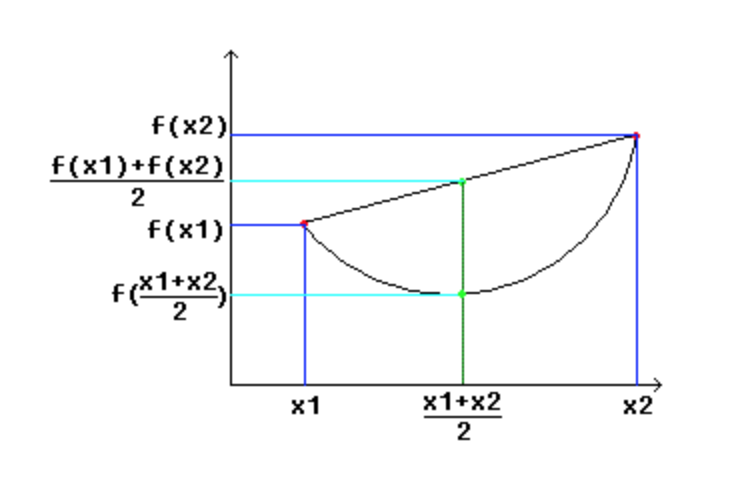定义3-凸函数的一般化定义：若f(x)$f\left(x\right)$$f(x)$为凸函数，x1,x2,…,xn${x}_{1},{x}_{2},\dots ,{x}_{n}$$x_1, x_2, \dots, x_n$是定义域D$D$$D$内的n$n$$n$个值，那么：

f(λ1x1+λ1x1+⋯+λnxn)≤λ1f(x1)+λ2f(x2)+⋯+λnf(xn)(9)$\begin{array}{}\text{(9)}& f\left({\lambda }_{1}{x}_{1}+{\lambda }_{1}{x}_{1}+\cdots +{\lambda }_{n}{x}_{n}\right)\le {\lambda }_{1}f\left({x}_{1}\right)+{\lambda }_{2}f\left({x}_{2}\right)+\cdots +{\lambda }_{n}f\left({x}_{n}\right)\end{array}$\begin{equation}
f(\lambda_1 x_1 + \lambda_1 x_1 + \dots + \lambda_n x_n) \leq \lambda_1 f(x_1) + \lambda_2 f(x_2) + \dots + \lambda_n f(x_n)
\end{equation}
其中，λi∈(0,1)${\lambda }_{i}\in \left(0,1\right)$$\lambda_i \in (0, 1)$，且∑ni=1λi=1$\sum _{i=1}^{n}{\lambda }_{i}=1$$\sum_{i = 1}^n \lambda_i = 1$。这也就是公式(2)中得到似然函数下界的依据。

4.2.2 凸函数的性质

下面给出凸函数的两个重要性质：

若f(x)$f\left(x\right)$$f(x)$在D$D$$D$上连续，且在D$D$$D$上二阶可导，则若f′′(x)>0${f}^{″}\left(x\right)>0$$f''(x) > 0$，f(x)$f\left(x\right)$$f(x)$为凸函数；反之，f(x)$f\left(x\right)$$f(x)$为凹函数
Jensen不等式：若f(x)$f\left(x\right)$$f(x)$是凸函数，则下面的公式(3)成立：

f((x1+x2+⋯+xn)/n)≤1n(f(x1)+f(x2)+⋯+f(xn))(3)$\begin{array}{}\text{(3)}& f\left(\left({x}_{1}+{x}_{2}+\cdots +{x}_{n}\right)/n\right)\le \frac{1}{n}\left(f\left({x}_{1}\right)+f\left({x}_{2}\right)+\cdots +f\left({x}_{n}\right)\right)\end{array}$\begin{equation}
f((x_1 + x_2 + \dots + x_n) / n) \leq \frac{1}{n} (f(x_1) + f(x_2) + \dots + f(x_n))
\end{equation}\tag{3}

可以看出，Jensen不等式就是凸函数的一般化定义的公式的一种特例（参数λi${\lambda }_{i}$$\lambda_i$全部取等了）。

放到概率论中，Jensen不等式是如下表述的。若f(x)$f\left(x\right)$$f(x)$为凸函数，则：

f(E(X))≥E(f(x))(4)$\begin{array}{}\text{(4)}& f\left(E\left(X\right)\right)\ge E\left(f\left(x\right)\right)\end{array}$\begin{equation}
f(E(X)) \geq E(f(x))
\end{equation}\tag{4}

公式(4)与公式(3)是一样的，只是在概率论中，把系数λi${\lambda }_{i}$$\lambda_i$的概念用概率替换了。需要注意的是，如果f(x)$f\left(x\right)$$f(x)$为严格凸函数，那么当且仅当变量X$X$$X$为常数时，即X=E(X)$X=E\left(X\right)$$X = E(X)$时，公式(4)的等号才成立。

4.3 E步求取隐藏变量的期望

了解了公式(4)，即Jensen不等式中等式成立的条件。我们回过头再看4.1节末尾的问题。此时使得lnL(θ)=J(z,Q)$\mathrm{ln}L\left(\theta \right)=J\left(z,Q\right)$$\ln L(\theta) = J(z, Q)$的条件就很明显了，因为J(z,Q)$J\left(z,Q\right)$$J(z, Q)$是变量p(xi,z(j);θ)Qi(z(j))$\frac{p\left({x}_{i},{z}^{\left(j\right)};\theta \right)}{{Q}_{i}\left({z}^{\left(j\right)}\right)}$$\frac{p(x_i, z^{(j)}; \theta)}{Q_i(z^{(j)})}$的期望（相当于E(f(x))$E\left(f\left(x\right)\right)$$E(f(x))$），那根据Jensen不等式，X$X$$X$为常数时，等号成立。

我们不妨设：
p(xi,z(j);θ)Qi(z(j))=c(10)$\begin{array}{}\text{(10)}& \frac{p\left({x}_{i},{z}^{\left(j\right)};\theta \right)}{{Q}_{i}\left({z}^{\left(j\right)}\right)}=c\end{array}$\begin{equation}
\frac{p(x_i, z^{(j)}; \theta)}{Q_i(z^{(j)})} = c
\end{equation}

其中c为常数。因为∑mj=1Qi(z(j))=1$\sum _{j=1}^{m}{Q}_{i}\left({z}^{\left(j\right)}\right)=1$$\sum_{j = 1}^m Q_i(z^{(j)}) = 1$，所以∑zp(xi,z(j);θ)=c$\sum _{z}p\left({x}_{i},{z}^{\left(j\right)};\theta \right)=c$$\sum_{z} p(x_i, z^{(j)}; \theta) = c$，有下式成立：

Qi(z(j))=p(xi,z(j);θ)∑mj=1p(xi,z(j);θ)=p(xi,z(j);θ)p(xi;θ)=p(z(j)|xi;θ)(5)$\begin{array}{}\text{(5)}& \begin{array}{rl}{Q}_{i}\left({z}^{\left(j\right)}\right)& =\frac{p\left({x}_{i},{z}^{\left(j\right)};\theta \right)}{\sum _{j=1}^{m}p\left({x}_{i},{z}^{\left(j\right)};\theta \right)}\\ & =\frac{p\left({x}_{i},{z}^{\left(j\right)};\theta \right)}{p\left({x}_{i};\theta \right)}\\ & =p\left({z}^{\left(j\right)}|{x}_{i};\theta \right)\end{array}\end{array}$\begin{equation}
\begin{aligned}
Q_i(z^{(j)}) &= \frac{p(x_i, z^{(j)}; \theta)}{\sum_{j = 1}^m p(x_i, z^{(j)}; \theta)}\\
&= \frac{p(x_i, z^{(j)}; \theta)}{p(x_i; \theta)}\\
&= p(z^{(j)}|x_i; \theta)
\end{aligned}
\end{equation}\tag{5}

其中p(z(j)|xi;θ)$p\left({z}^{\left(j\right)}|{x}_{i};\theta \right)$$p(z^{(j)}|x_i; \theta)$是为zi${z}_{i}$$z_i$的后验概率。公式(5)解决了如何选择Q(z)$Q\left(z\right)$$Q(z)$能使得似然函数与其下界相等的问题，其实就是令Qi(z(j))${Q}_{i}\left({z}^{\left(j\right)}\right)$$Q_i(z^{(j)})$为z(j)${z}^{\left(j\right)}$$z^{(j)}$的后验概率即可。实际操作中，这个后验概率是根据初始的分布参数决定的。这一步就是EM算法中的E步，Qi(z(j))${Q}_{i}\left({z}^{\left(j\right)}\right)$$Q_i(z^{(j)})$就是隐藏变量的期望。

4.4 M步求解最大似然函数

跟4.1节介绍的方法一致，现在既然

lnL(θ)=∑i=1n∑j=1mQi(z(j))lnp(xi,z(j);θ)Qi(z(j))(11)$\begin{array}{}\text{(11)}& \mathrm{ln}L\left(\theta \right)=\sum _{i=1}^{n}\sum _{j=1}^{m}{Q}_{i}\left({z}^{\left(j\right)}\right)\mathrm{ln}\frac{p\left({x}_{i},{z}^{\left(j\right)};\theta \right)}{{Q}_{i}\left({z}^{\left(j\right)}\right)}\end{array}$\begin{equation}
\ln L(\theta) = \sum_{i = 1}^n \sum_{j = 1}^m Q_i(z^{(j)}) \ln \frac{p(x_i, z^{(j)}; \theta)}{Q_i(z^{(j)})}
\end{equation}

那就对这个函数关于θ$\theta$$\theta$求偏导，令偏导数为0即可。然后迭代执行E步和M步，直到收敛。

EM算法的E步和M步可以形式化的表示如下：

E步：Qi(z(j))=p(xi,z(j);θ)${Q}_{i}\left({z}^{\left(j\right)}\right)=p\left({x}_{i},{z}^{\left(j\right)};\theta \right)$$Q_i(z^{(j)}) = p(x_i, z^{(j)}; \theta)$
M步：θ=maxθ∑ni=1∑mj=1Qi(z(j))lnp(xi,z(j);θ)Qi(z(j))$\theta =ma{x}_{\theta }\sum _{i=1}^{n}\sum _{j=1}^{m}{Q}_{i}\left({z}^{\left(j\right)}\right)\mathrm{ln}\frac{p\left({x}_{i},{z}^{\left(j\right)};\theta \right)}{{Q}_{i}\left({z}^{\left(j\right)}\right)}$$\theta = max_{\theta} \sum_{i = 1}^n \sum_{j = 1}^m Q_i(z^{(j)}) \ln \frac{p(x_i, z^{(j)}; \theta)}{Q_i(z^{(j)})}$

5. EM算法的收敛性证明

但是我们写到这里还有一个疑问，这种反复迭代一定会收敛吗？假定θ(t)${\theta }^{\left(t\right)}$$\theta^{(t)}$和θ(t+1)${\theta }^{\left(t+1\right)}$$\theta^{(t + 1)}$为第t$t$$t$轮和第t+1$t+1$$t + 1$轮迭代后的结果，l(θ(t))$l\left({\theta }^{\left(t\right)}\right)$$l(\theta^{(t)})$和l(θ(t+1))$l\left({\theta }^{\left(t+1\right)}\right)$$l(\theta^{(t + 1)})$为对应的似然函数。显然，如果l(θ(t))≤l(θ(t+1))$l\left({\theta }^{\left(t\right)}\right)\le l\left({\theta }^{\left(t+1\right)}\right)$$l(\theta^{(t)}) \leq l(\theta^{(t + 1)})$，那么随着迭代次数的增加，最终会一步步逼近最大似然值。也就是说，只需要证明公式(6)成立即可。

l(θ(t))<l(θ(t+1))(6)$\begin{array}{}\text{(6)}& l\left({\theta }^{\left(t\right)}\right)\begin{equation}
l(\theta^{(t)}) < l(\theta^{(t + 1)})
\end{equation}\tag{6}

证明：得到θ(t)${\theta }^{\left(t\right)}$$\theta^{(t)}$后，执行E步：

Qti(z(j))=p(z(j)|xi;θt)(20)$\begin{array}{}\text{(20)}& {Q}_{i}^{t}\left({z}^{\left(j\right)}\right)=p\left({z}^{\left(j\right)}|{x}_{i};{\theta }^{t}\right)\end{array}$\begin{equation}
Q_i^{t}(z^{(j)}) = p(z^{(j)}|x_i; \theta^{t})
\end{equation}

此时，

l(θ(t))=∑i=1n∑j=1mQi(z(j))lnp(xi,z(j);θt))Qi(z(j))(22)$\begin{array}{}\text{(22)}& l\left({\theta }^{\left(t\right)}\right)=\sum _{i=1}^{n}\sum _{j=1}^{m}{Q}_{i}\left({z}^{\left(j\right)}\right)\mathrm{ln}\frac{p\left({x}_{i},{z}^{\left(j\right)};{\theta }^{t}\right)\right)}{{Q}_{i}\left({z}^{\left(j\right)}\right)}\end{array}$\begin{equation}
l(\theta^{(t)}) =\sum_{i = 1}^n \sum_{j = 1}^m Q_i(z^{(j)}) \ln \frac{p(x_i, z^{(j)}; \theta^{t}))}{Q_i(z^{(j)})}
\end{equation}

然后执行M步，求偏导为0，得到θ(t+1)${\theta }^{\left(t+1\right)}$$\theta^{(t + 1)}$，此时有公式(7)成立：

l(θ(t+1))≥∑i=1n∑j=1mQi(z(j))lnp(xi,z(j);θ(t+1)))Qi(z(j))≥∑i=1n∑j=1mQi(z(j))lnp(xi,z(j);θt))Qi(z(j))=l(θt)(7)$\begin{array}{}\text{(7)}& \begin{array}{rl}l\left({\theta }^{\left(t+1\right)}\right)& \ge \sum _{i=1}^{n}\sum _{j=1}^{m}{Q}_{i}\left({z}^{\left(j\right)}\right)\mathrm{ln}\frac{p\left({x}_{i},{z}^{\left(j\right)};{\theta }^{\left(t+1\right)}\right)\right)}{{Q}_{i}\left({z}^{\left(j\right)}\right)}\\ & \ge \sum _{i=1}^{n}\sum _{j=1}^{m}{Q}_{i}\left({z}^{\left(j\right)}\right)\mathrm{ln}\frac{p\left({x}_{i},{z}^{\left(j\right)};{\theta }^{t}\right)\right)}{{Q}_{i}\left({z}^{\left(j\right)}\right)}=l\left({\theta }^{t}\right)\end{array}\end{array}$\begin{equation}
\begin{aligned}
l(\theta^{(t + 1)}) &\geq \sum_{i = 1}^n \sum_{j = 1}^m Q_i(z^{(j)}) \ln \frac{p(x_i, z^{(j)}; \theta^{(t + 1)}))}{Q_i(z^{(j)})}\\
& \geq \sum_{i = 1}^n \sum_{j = 1}^m Q_i(z^{(j)}) \ln \frac{p(x_i, z^{(j)}; \theta^{t}))}{Q_i(z^{(j)})} = l(\theta^{t})
\end{aligned}
\end{equation}\tag{7}

简单说一下公式(7)，第一步l(θ(t+1))≥∑ni=1∑mj=1Qi(z(j))lnp(xi,z(j);θ(t+1))Qi(z(j))$l\left({\theta }^{\left(t+1\right)}\right)\ge \sum _{i=1}^{n}\sum _{j=1}^{m}{Q}_{i}\left({z}^{\left(j\right)}\right)\mathrm{ln}\frac{p\left({x}_{i},{z}^{\left(j\right)};{\theta }^{\left(t+1\right)}\right)}{{Q}_{i}\left({z}^{\left(j\right)}\right)}$$l(\theta^{(t + 1)}) \geq \sum_{i = 1}^n \sum_{j = 1}^m Q_i(z^{(j)}) \ln \frac{p(x_i, z^{(j)}; \theta^{(t + 1)})}{Q_i(z^{(j)})}$是由前面的公式(2)决定的；

第二步  ≥∑ni=1∑mj=1Qi(z(j))lnp(xi,z(j);θt))Qi(z(j))$\ge \sum _{i=1}^{n}\sum _{j=1}^{m}{Q}_{i}\left({z}^{\left(j\right)}\right)\mathrm{ln}\frac{p\left({x}_{i},{z}^{\left(j\right)};{\theta }^{t}\right)\right)}{{Q}_{i}\left({z}^{\left(j\right)}\right)}$$\geq \sum_{i = 1}^n \sum_{j = 1}^m Q_i(z^{(j)}) \ln \frac{p(x_i, z^{(j)}; \theta^{t}))}{Q_i(z^{(j)})}$是M步的定义，M步中，将θt${\theta }^{t}$$\theta^{t}$调整到θ(t+1)${\theta }^{\left(t+1\right)}$$\theta^{(t + 1)}$就是为了使似然函数l(θ(t+1))$l\left({\theta }^{\left(t+1\right)}\right)$$l(\theta^{(t + 1)})$最大化。

综上，我们证明了EM算法的收敛性。
展开全文最大似然估计 Jensen不等式
• ·窗口最大化快捷键　Shift+WIND+M Windows键+CTRL+M功能是：重新将恢复上一项操作前窗口的大小和位置 ·在最大化和最小化之间切换的快捷键：WIN＋D ·　最大化ALT+空格+X 　最小化ALT+空格+N 其它有关...
 最小化的快捷键　WIN＋M
按“Windows键+M”键可以 最小化所有被打开的窗口，相当于“显示桌面”功能。
·窗口最大化快捷键　Shift+WIND+M
Windows键+CTRL+M功能是：重新将恢复上一项操作前窗口的大小和位置
·在最大化和最小化之间切换的快捷键：WIN＋D

·　最大化ALT+空格+X
最小化ALT+空格+N
其它有关WINDOWS键的快捷键

Windows键+E 打开资源管理器
Windows键+F 打开“查找：所有文件”对话框

Windows键+R 打开“运行”对话框
Windows键+BREAK 打开“系统属性”对话框
Windows键+CTRL+F打开“查找：计算机”对话框
SHIFT+F10或鼠标右击 打开当前活动项目的快捷菜单
WINDOWS+F1 显示“帮助”
WINDOWS+TAB 在任务栏上的按钮间循环
ALT+TAB 切换当前程序

有关F系列快捷键
在WINDOWS系统下的作用：
F1 显示当前程序或者Windows的帮助内容。
F2 当你选中一个文件的话，这意味着“重命名”
F3 当你在桌面上的时候是打开“查找：所有文件” 对话框
F5　WINDOWS操作中，显示“查找：所有文件” 。网页浏览器中按F5键为刷新，CTRL+F5为强制刷新缓存
F4　需与其它键配合使用，（在IE中按F4或F6键可以在地址栏中弹出下拉菜单供选择或输入网址，ALT＋D可以选择地址栏）
ALT+F4 关闭当前窗口或退出程序
CTRL+F4 关闭当前应用程序中的当前文本（如word中）
CTRL+F6 切换到当前应用程序中的下一个文本（加shift 可以跳到前一个窗口）
F10或ALT激活当前程序的菜单栏 IE7浏览器的快捷键

一般快捷键
打开/关闭全屏模式 —— F11
循环的选择地址栏，刷新键和当前标签页 —— TAB
在当前标签页查询字或短语 —— CTRL+F
为当前标签页打开一个新窗口 —— CTRL+N
打印当前标签页 —— CTRL+P
选择当前页的所有内容 —— CTRL+A
放大 —— CTRL+Plus（由于前面是加号为避免误解所以用Plus代表“+”）
缩小 —— CTRL+-
恢复原始大小 —— CTRL+0
导航快捷键
返回主页 —— ALT+HOME
返回后一页 —— ALT+LEFT
返回前一页 —— ALT+RIGHT
刷新 —— F5
刷新页面同时刷新缓存 —— CTRL+F5
停止下载页面 —— ESC
收藏夹中心快捷键
打开收藏夹 —— CTRL+I
以固定模式打开收藏夹 —— CTRL+SHIFT+I
整理收藏夹 —— CTRL+B
将当前页添加到收藏夹 —— CTRL+D
打开 Feeds —— CTRL+J
以固定模式打开 Feeds —— CTRL+SHIFT+J
打开历史 —— CTRL+H
以固定模式打开历史 —— CTRL+SHIFT+H
标签快捷键
用新标签打开链接并切换至新标签 —— CTRL+ 鼠标左键 or 鼠标中键
用新标签打开链接但不切换至新标签 —— CTRL+SHIFT+ 鼠标左键 or CTRL+SHIFT+ 鼠标中键
关闭当前标签页（如果只有一个标签将关闭IE） —— CTRL+W or CTRL+F4
打开快速标签视图 —— CTRL+Q
打开一个新标签 —— CTRL+T
查看打开标签的列表 —— CTRL+SHIFT+Q
切换到下一个标签 —— CTRL+TAB
切换到前一个标签 —— CTRL+SHIFT+TAB
地址栏快捷键
选择地址栏 —— ALT+D
在地址栏中的文本初出添加"http://www." 和末尾添加".com" —— CTRL+ENTER
在地址栏中的文本初出添加"http://www." 和末尾添加你自定义的后缀 —— CTRL+SHIFT+ENTER
用新标签打开地址栏的网址 —— ALT+ENTER *
查看以前出入的地址 —— F4
搜索栏快捷键
选择搜索栏 —— CTRL+E
查看搜索引擎列表 —— CTRL+DOWN
用新标签打开搜索结果 —— ALT+ENTER
一些设置操作
禁用所有插件 —— Windows logo key + R > iexplore.exe –extoff
开启自动完成功能这样网址在你再一次输入它们的时候会自动完成 —— Tools > Internet Options > Advanced > Use inline AutoComplete

改变Internet Explorer的标签设置 —— Tools > Internet Options > 在General tab里, Tabs下, 点击Settings

在屏幕上方显示菜单栏 —— 临时显示: 按 ALT 键
删除 s, 密码, 表单数据, 历史, and 临时文件 —— Tools > Delete Browsing History
打印页面部分内容 —— 选择你想打印的部分, 按 CTRL+P, 点击 Selection, 然后按 Print
改变网址后缀 —— Tools > Internet Options > Languages
* - 例如，如果你选择"net"作为网址后缀，按下 CTRL+SHIFT+ENTER 将会添加"http://www." 和".net"的后缀。

另外如果网页显示的文字大小不正常，可以选择“查看”/"文字大小"/然后，选择最大，最小，适中等字体就可以了，

展开全文windows internet tools
• ## 期望值最大化算法

千次阅读 2015-12-12 19:54:36
在参数估计中常常通过最大似然函数进行估计，由于隐变量的存在，不能直接求解这个最大似然函数，期望值最大化算法就是将这个最大似然函数的求解问题转化为求解其下界的最大值的问题，通过一个求隐变量的分布的“期望...
一，最大似然估计与隐变量
期望值最大化算法是用来对包含隐变量的样本点的分布函数的参数估计方法。在参数估计中常常通过最大似然函数进行估计，由于隐变量的存在，不能直接求解这个最大似然函数，期望值最大化算法就是将这个最大似然函数的求解问题转化为求解其下界的最大值的问题，通过一个求隐变量的分布的“期望值”步骤和一个求似然函数最大化的“最大值”步骤完成。
假如有一批训练样本Xi （i=1,2,..I），这批样本服从参数为 \theta 的分布，要估计 \theta的值，可以通过求解如下似然函数的最大值进行：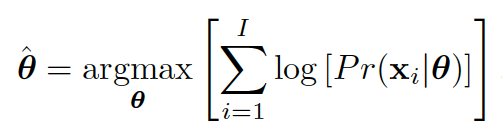（1）
如果Xi的分布函数仅仅依赖于\theta, 就可以写出 p(x|theda)的表达式，这个最优化问题可以直接求解。
现在的情况是Xi的分布函数不仅依赖于\theta，还依赖于另一个变量h, 每一个样本Xi都对应着h的一个值，h本身服从一个未知的分布。实际上 x和h的联合分布是以theta作为参数的，而p(x|theda)是这个联合分布的边缘分布：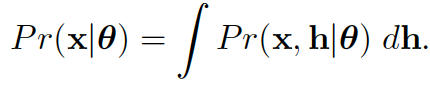（2）
因此现在的似然函数就变为：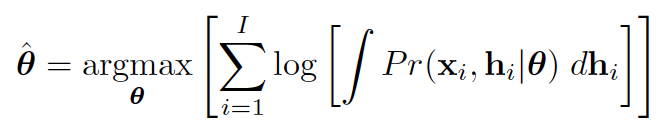（3）
二，期望值最大化的原理
期望最大化求解上述似然函数的中心思想是为了回避直接求解这个函数的困难，转而求解它的一个下界函数B(theda)的最大值。这个下界函数要保证始终不大于原函数，并且通过精心的选择，以使得求解这个下界函数的最优化参数相当容易。考虑到隐变量h的分布函数qi(hi)未知，要估计的参数theta未知，这个下界函数是关于qi(hi)和theta的函数，其定义如下：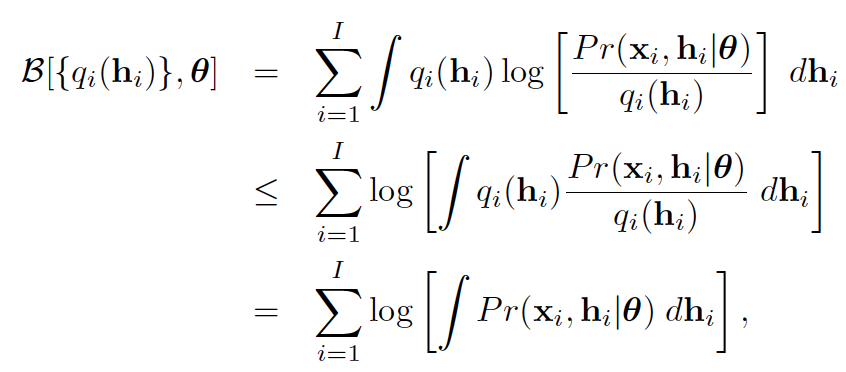（4）
上面的不等式是根据Jensen不等式得出的。Jensen不等式为E(f(x))=<f(E(x)),其中E()表示期望值，f(x)是一个凹函数，因为log函数是一个凹函数，可推导出上式。
得到这个下界函数后要使其最大化，分为两个迭代的步骤进行，第一个步骤称为“期望值（E）”步骤，求qi(hi)的最优化参数，第二个步骤称为“最大化”（M)步骤，求theta的最优化参数。
E步骤
对公式（4）进行变换可得，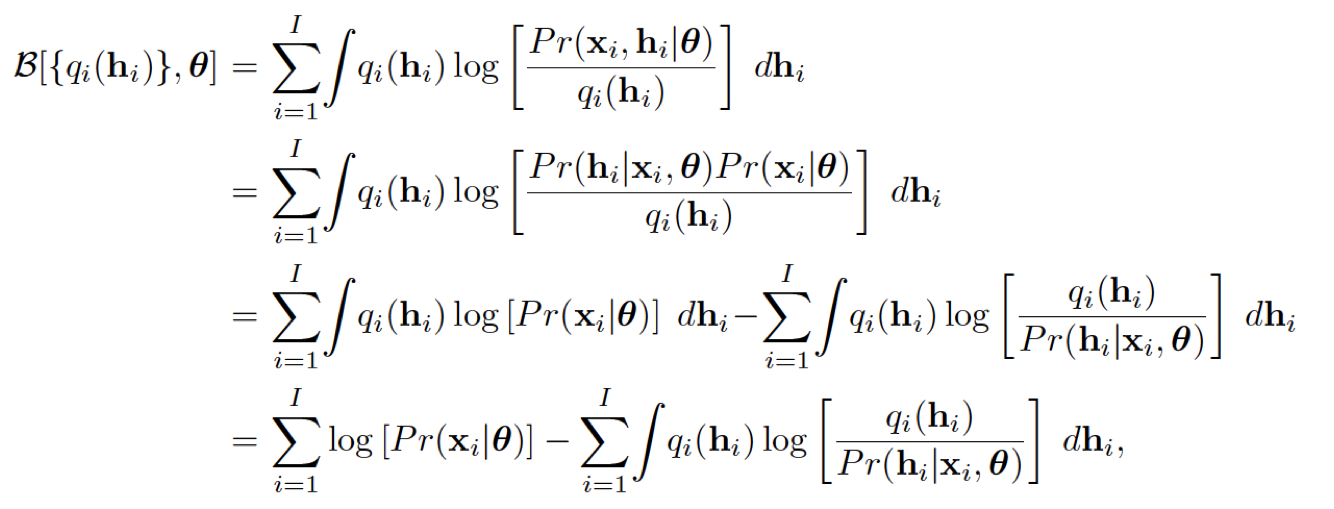（5）
其中第一项与qi(hi)无关，因此qi(hi)的最优化参数为：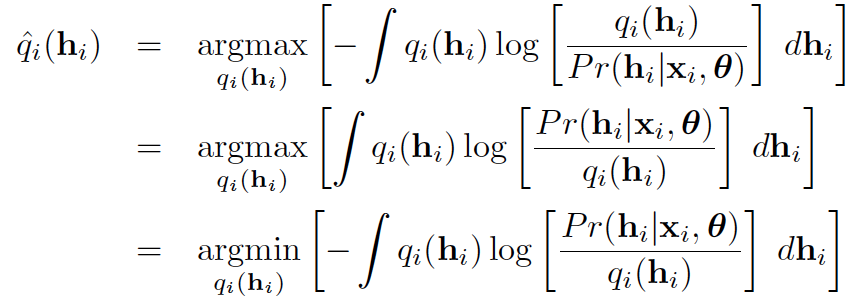（6）
上式把最大化问题转化为最小化问题。根据log(y)<=y-1，可得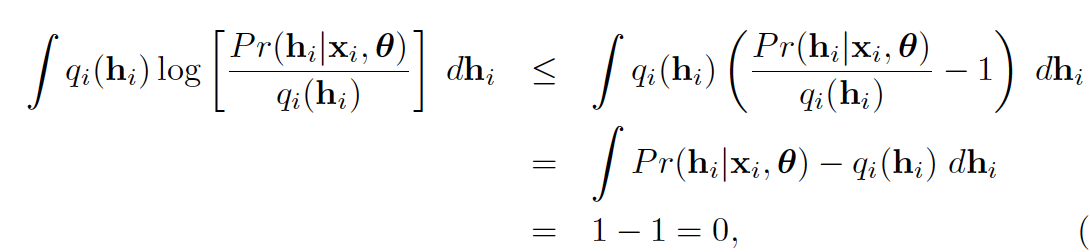7）
上式取得最优值即等号满足的条件是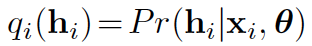，在给定的theta和Xi的条件下计算方法如下：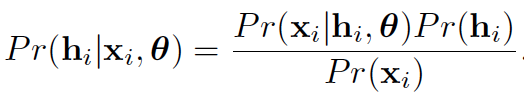（8）
利用上式对每一个样本的隐变量hi所有可能存在的取值进行计算，可得到这些隐变量的分布函数。其中分母通过全概率公式计算得到。
M步骤
这一步算theta的最优值。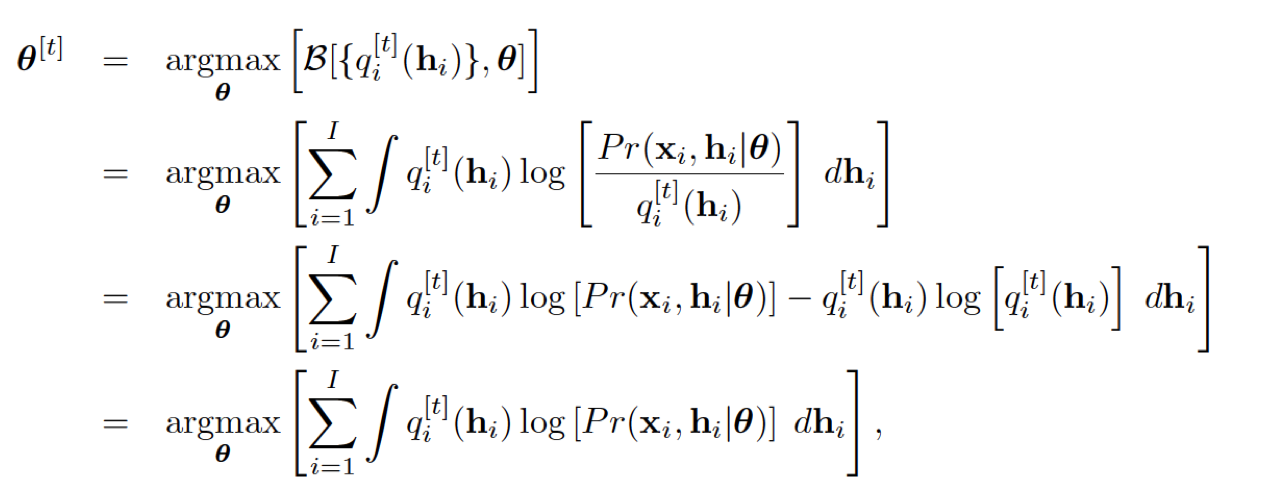（9）
上式再根据具体的问题中xi与hi的联合密度函数展开求最优值。
三，高斯混合模型的参数估计
高斯混合模型的参数为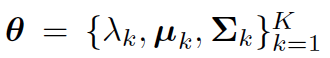，分别是各个高斯分布的比重，均值和方差，可通过EM进行估计。这里的隐变量的含义就是各个样本所属的高斯分布编号即类别，每一个类别表示一个高斯分布。在所得到的样本中只知道各样本的特征值xi,而不知道该样本所属的类别hi。此处的问题就是如何利用这些未知类别标号的样本来估计高斯混合分布的参数。
在E步骤中，计算各个样本分别属于各个类别的概率，得到该样本的类别，即隐变量的分布函数：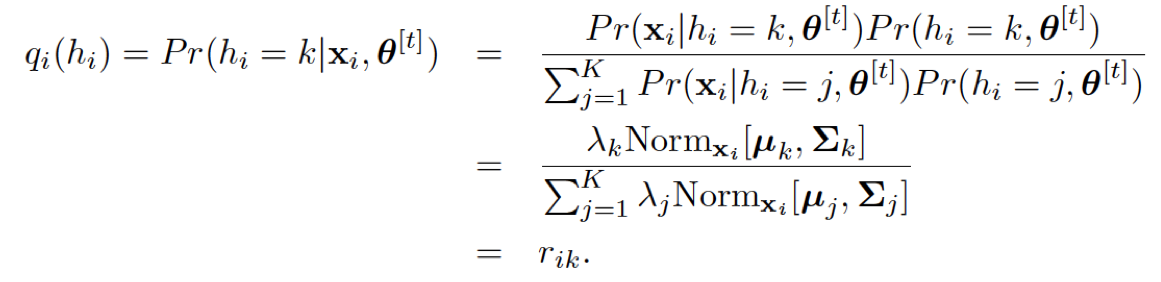（10）
在M步骤中，求参数的最优值，公式（9）变为：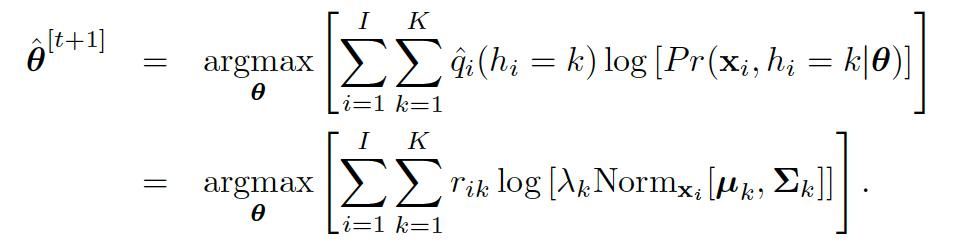（11）
可以通过对参数求导得到其最优值，结果为：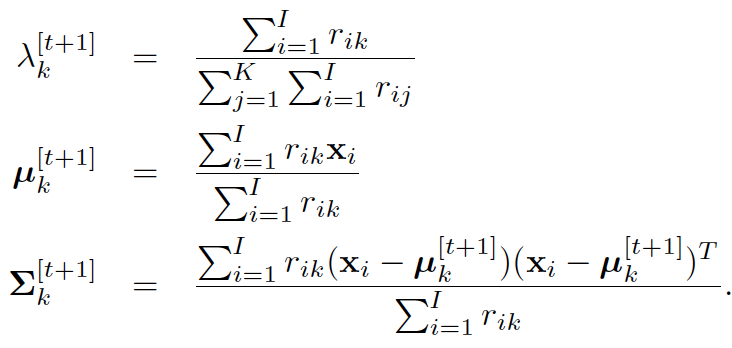（12）
以上E和M步骤交替进行，直到收敛。


展开全文• 注意:ELM构造分类器得到的结果并不在(0,1)区间,通过软最大化将分类结果整合到(0,1)区间,通过模糊积分判别样本的结果. 具体实现步骤: 对于多个ELM分类器软最大化到模糊积分的具体实现: 软最大化具体实现: ...
核心思想:
非平衡数据集—->转化成平衡数据集—->ELM构造分类器—–>软最大化—–>模糊积分
注意:ELM构造分类器得到的结果并不在(0,1)区间,通过软最大化将分类结果整合到(0,1)区间,通过模糊积分判别样本的结果.
具体实现步骤: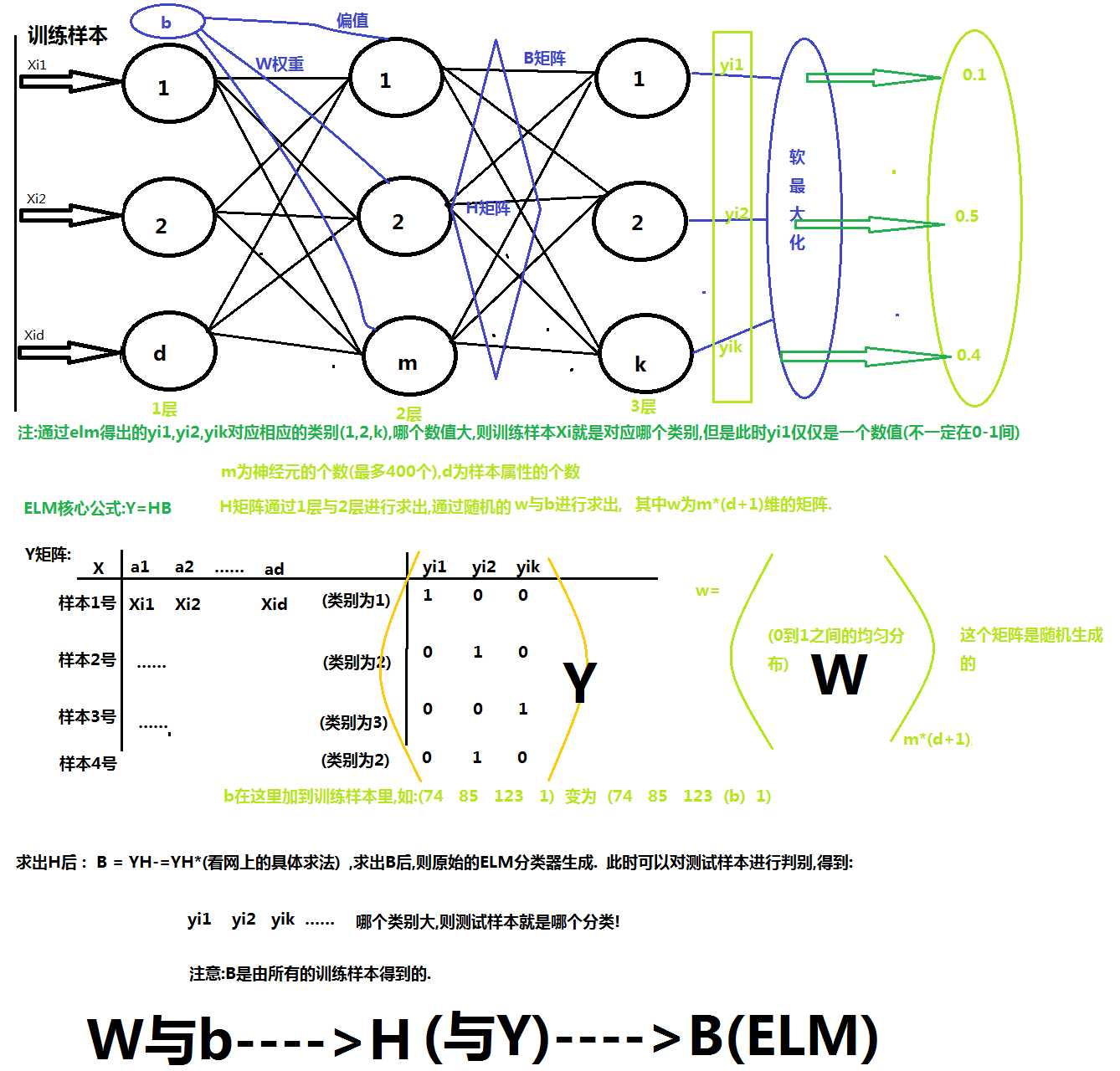对于多个ELM分类器软最大化到模糊积分的具体实现: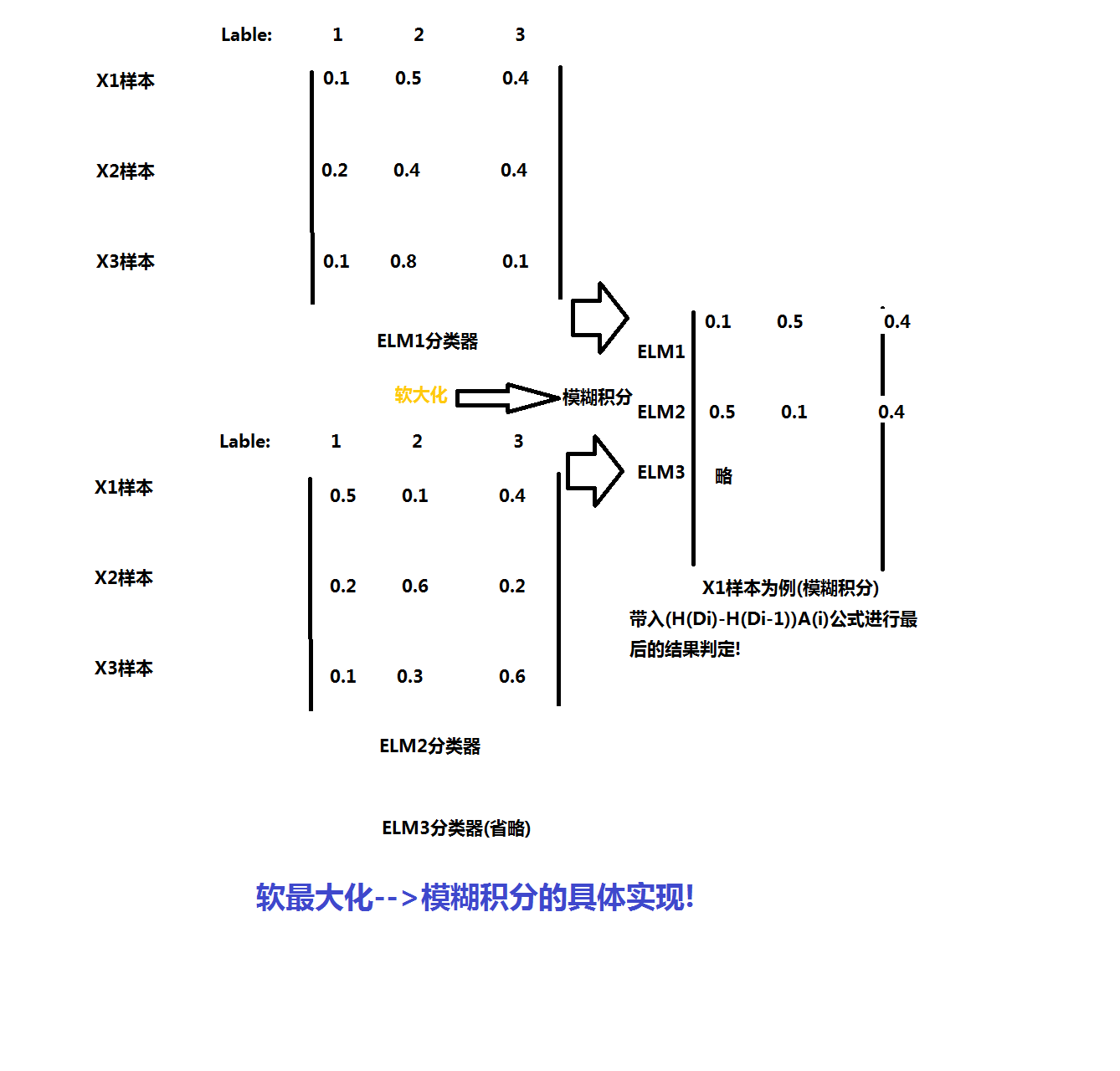软最大化具体实现: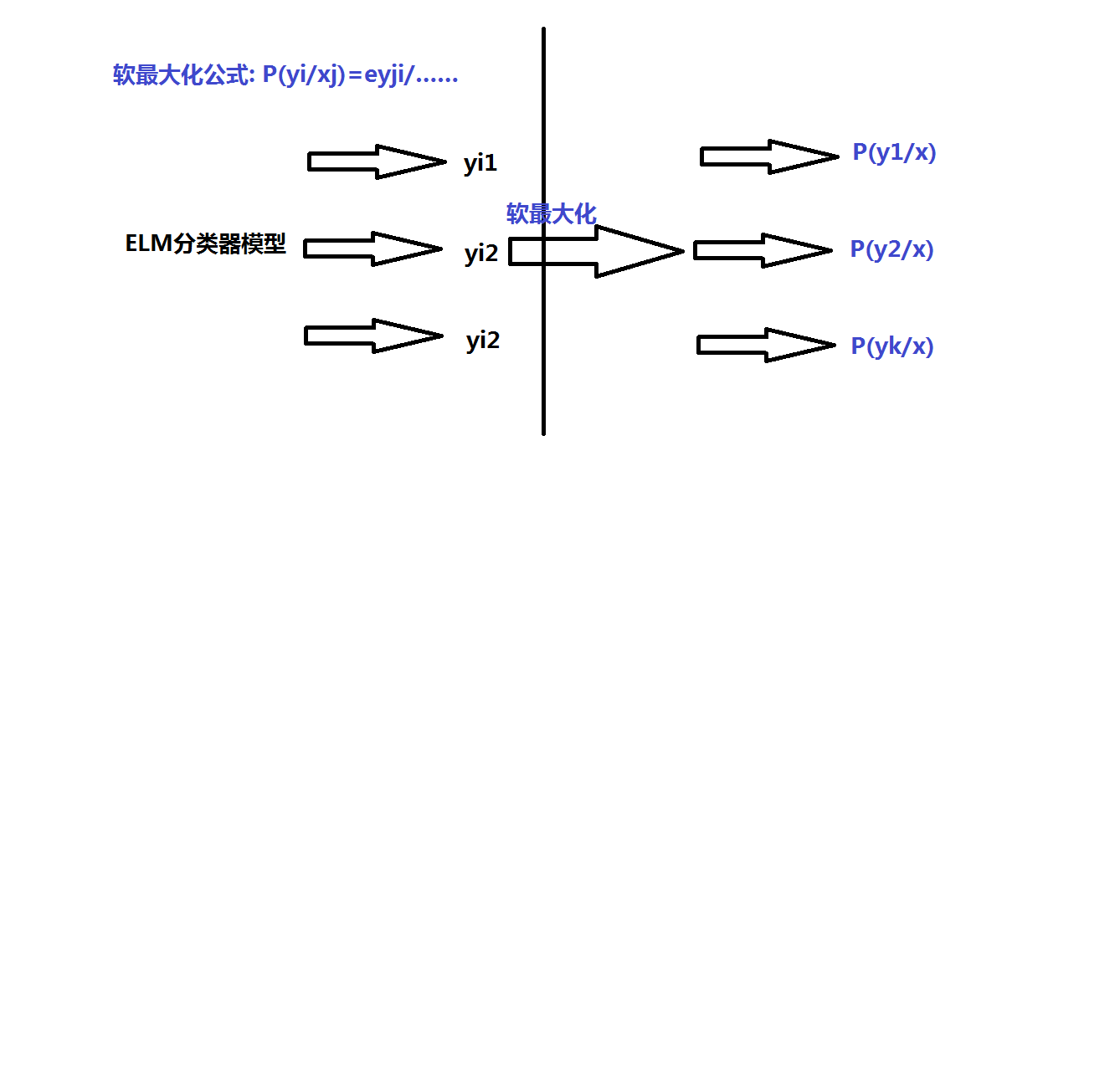模糊积分具体实现: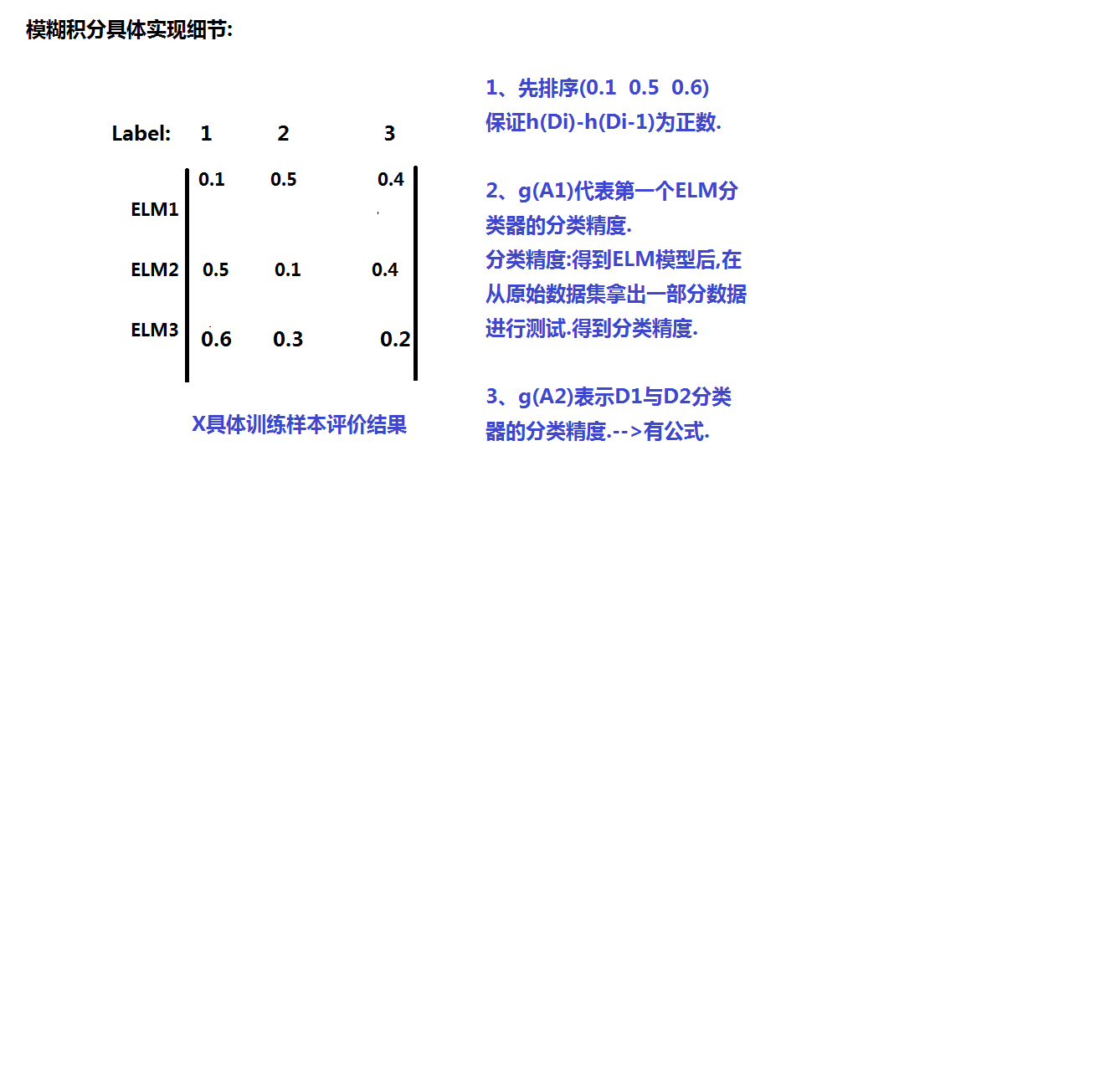展开全文ELM 模糊积分
• ## EM-期望最大化算法

千次阅读 2015-11-04 21:01:55
最大期望算法（Expectation Maximization Algorithm，又译期望最大化算法），是一种迭代算法，用于含有隐变量（hidden variable）的概率参数模型的最大似然估计或极大后验概率估计。 中文名 em算法 外文名 ...算法
• ## Java实现币值最大化问题

万次阅读 多人点赞 2019-07-21 21:24:11
请问如何选择硬币，使得在其原始位置互不相邻的条件下，所选硬币的总金额最大。 2 解决方案 2.1 动态规划法 本文所写代码思想参考自《算法设计与分析基础》第三版上一段讲解，具体如下： package ...Java
• 币值最大化 问题描述 给定一排n个硬币，其面值均为整数c1, c2, …, cn, 这些整数并不一定两两不同。问如何选择硬币，使得在其原始位置互不相邻的条件下，所选硬币的总金额最大。 解题思路 上述最大可选金额用f(n...
• 对于影响力最大化问题，我以前写过两个blog 影响力最大化 IC模型+贪心算法 影响力最大化 模拟爆发(粗糙笔记) 但是，对于这两个方法都不是最优的： 对于IC模型 模型使用了贪心算法，然后遍历激活结点，每一次遍历将...算法 python
• 这几天在看QT，虽然QT已经广泛使用了这么多年，但是自己还是第一次用，遇到许多问题，QT窗体的最大化最小化就是其中之一。下面我说一下。  虽然QT中提供了最大化showMaximized()函数，和全屏函数showFullScreen()...qt 任务
• 起初看的是这篇文章，结果发现这个根本不行：http://blog.csdn.net/ziren235/archive/2006/05/19/745651.aspx在程序运行过程中可明显看到窗口时生成后最大化的，有个最大化的过程～～还要找找原因终于找到了解决方法...
• ## MFC窗口启动最大化

千次阅读 2012-05-23 12:22:04
常用MFC进行编程的人都知道单文档的编程，在编程过程中发现欲使程序在启动时就能最大化显示，这里的处理方法有多种，但是结果却不一样。 首先大家最常用的就是在View类的PreCreateWindow函数中，修改窗口类型cs的值...command shell 文档
• 最大化 LoRa 长距离，秘籍1：发射功率 The prudent are crowned with knowledge.（通达人得知识为冠冕）-- 《旧·箴》14：18 引言 正如《最大化 LoRa 长距离，您应该知道的完整秘籍》，提高发射功率可有效延长...LoRaWAN
• 2浏览器最大化 3截屏并保存文件 4退出浏览器 二、代码 #coding:utf-8 ''' 1设置浏览器窗口大小 2浏览器最大化 3截屏并保存文件 4退出浏览器 ''' #导入依懒的模块 from selenium import webdriver as web ...
• var hwnd: hwnd;//句柄 PostMessage(hwnd,WM_SYSCOMMAND, SC_MINIMIZE,0); //最小化 PostMessage(hwnd,WM_...//最大化 PostMessage(hwnd,WM_SYSCOMMAND, SC_CLOSE,0);//关闭 窗口最小化时将释放占用的资源delphi
• ## 影响力最大化 IC模型+贪心算法

千次阅读 热门讨论 2020-01-28 20:39:07
记录一下影响力最大化的遍历解决的方法，写的很粗糙，就是怕自己以后忘了，不喜勿喷。 一起共勉~~ 贪心 （1）首先|S|=1，在所有点中选一个在IC模型下跑出感染的点数量最多的点加入S （此时跑了n趟IC） （2）再在剩下...算法 python 数据结构
• 社交网络影响力最大化——贪心算法实现（Python实现） １、环境配置 环境： Win7 Pycharm Anaconda2 该算法每个节点的阈值设为 0.5 ２、LT传播模型算法实现 linear_threshold_clime.py（LT传播模型算法） #...社交网络 贪心算法
• 1.在建立frame的时候使用wxMAXIMIZE参数,不可以和caption属性一起用，建立出来的最大化窗口会没有标题栏,最大最小化按钮等东 西,属于工作区的最大化; 2.在显示窗口的时候一般为使用Show(true),可以选择使用...windows 文档窗体显示问题
• 有的时候定制窗口，隐藏系统窗口的标题栏，自己绘制最大最小化和关闭按钮，这样当窗口最大化时会发现最顶部一小部分超出了屏幕区域 解决方法是处理WM_GETMINMAXINFO消息...
• 社交网络影响力最大化——线性阈值模型（LT模型）算法实现（Python实现） １、环境配置 环境配置：Win7 Pycharm Anaconda2 该算法每个节点的阈值设为 0.5 ２、LT传播模型算法实现 linear_threshold.py (L....社交网络 线性阈值模型
• ## 最大最小化标准化

万次阅读 2019-02-28 21:10:47
不同评价指标往往具有不同的量纲，数值见的差别可能很大，不进行处理可能会影响到数据分析的结果。为了消除指标之间的量纲和取值范围差异的影响，需要进行标准处理，将数据按照比例进行缩放，使之落入一个特定的...
• 场景描述： 程序有一个MDI父窗体frmMain，内含一个MenuStrip控件mnMain MDI子窗体名为frmTransfer mnMain包含菜单项“运单管理”及其子菜单项“调件运单” 当点击“购件运单”菜单项时，要求自动以最大化的方式显示...button object system windows string
• 编程时遇到一个问题：WebBrowser控件加载添加收件人页面时（弹出页面），WebBrowser控件显示区域很小，导致无法看到全部页面，并且和最大化后的窗体之间留有很大的空白区域。解决：Private Sub Form_Resize()...webbrowser object 测试 url
• 在该MFC项目中Ctrl+F，查找EXSTYLE WS_EX_APPWINDOW，查找结果在 项目名.rc 文件中，如： IDD_TESTMFC_DIALOG DIALOGEX 0, 0, 320, 200 STYLE DS_SHELLFONT | WS_POPUP | WS_VISIBLE | WS_CAPTION  | WS_...
• 社交网络中基于位置的影响力最大化 摘要 这篇文章的目的是通过研究在LBSN平台中基于位置的影响最大化来实现O2O模式上的产品推广。随着O2O环境下存在的消费行为，传统的线上影响力扩散模型不能准确描述产品验收过程。...社交网络 算法
• 最后打印结果： 股票价格 [77, 84, 59, 56, 69, 38, 53, 77, 35, 89] -------------------- 股票价格差值 [7, -25, -3, 13, -31, 15, 24, -42, 54] 股票增值数 [7, 13, 15, 24, 54] 股票最大收益 113python python面试 python计算股票
• 最大化最大似然估计，也就是    这是将 的k种情况展开后的样子，未知参数 和 。  固定 和 ，对 求导得    等于0时，得到    这就是我们之前模型中的 的更新公式。 ...
• 一般而言最大化最小值问题都会只有一个确定的答案（如果有多个答案，也应该都是一样的值）这是显然的。那么在二分过程中我们应该是不断缩小[L，R]的范围，最终使得L==R才退出二分。这样才是一个唯一的结果。如果当......